Fingerings Home Octave 1: F  (C) Gb  (Db) G  (D) Ab  (Eb) A  (E) Bb  (F) B  (Gb) C  (G) Db  (Ab) D  (A) Eb  (Bb) E  (B) Octave 2+: F  (C) Gb  (Db) G  (D) Ab  (Eb) A  (E) Bb  (F) B  (Gb) C  (G) Db  (Ab) D  (A) Eb  (Bb) E  (B) F  (C) Gb  (Db) G  (D)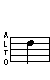D (A) - 1st Octave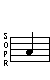## Trills

Trill - From E
(See below for alternate)
Trill - From Eb
(See below for alternate)
E D   Eb D (alt)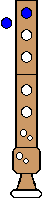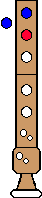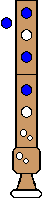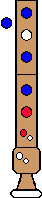Alternate Trill - From E   Alternate Trill - From Eb
E D (alt)   Eb (alt) D (alt)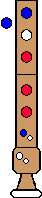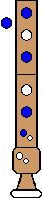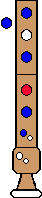Comments: Exactly why you would ever use this is unclear :-)   Comments:
Top

## Turned Trills

Turned Trill
Full step above, Full step below
E D C D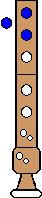Top

Turned Trill
Half step above; Full step below
Eb D (alt) C DTop

Turned Trill
Full step above; Half step below
E D Db D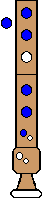Top

Turned Trill
Half step above; Half step below
Eb D (alt) Db DTop

## Regular Turns

Turn
Full step above; Full step below
E D C DTop

Turn
Half step above; Full step below
Eb D C DTop

Turn
Full step above; Half step below
E D Db D